The FREQ Procedure

### Odds Ratio and Relative Risks for 2 x 2 Tables

#### Odds Ratio (Case-Control Studies)

The odds ratio is a useful measure of association for a variety of study designs. For a retrospective design called a case-control study, the odds ratio can be used to estimate the relative risk when the probability of positive response is small (Agresti 2002). In a case-control study, two independent samples are identified based on a binary (yes-no) response variable, and the conditional distribution of a binary explanatory variable is examined, within fixed levels of the response variable. See Stokes, Davis, and Koch (2000) and Agresti (2007).

The odds of a positive response (column 1) in row 1 is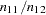. Similarly, the odds of a positive response in row 2 is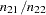. The odds ratio is formed as the ratio of the row 1 odds to the row 2 odds. The odds ratio for a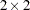table is defined as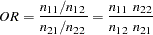The odds ratio can be any nonnegative number. When the row and column variables are independent, the true value of the odds ratio equals 1. An odds ratio greater than 1 indicates that the odds of a positive response are higher in row 1 than in row 2. Values less than 1 indicate the odds of positive response are higher in row 2. The strength of association increases with the deviation from 1.

The transformation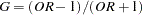transforms the odds ratio to the range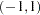with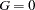when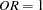;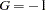when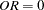; and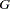approaches 1 as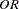approaches infinity.is the gamma statistic, which PROC FREQ computes when you specify the MEASURES option.

The asymptotic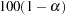% confidence limits for the odds ratio are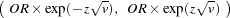where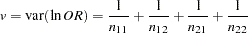andis theth percentile of the standard normal distribution. If any of the four cell frequencies are zero, the estimates are not computed.

When you specify the OR option in the EXACT statement, PROC FREQ computes exact confidence limits for the odds ratio. Because this is a discrete problem, the confidence coefficient for the exact confidence interval is not exactly (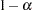) but is at least (). Thus, these confidence limits are conservative. See Agresti (1992) for more information.

PROC FREQ computes exact confidence limits for the odds ratio by using an algorithm based on Thomas (1971). See also Gart (1971). The following two equations are solved iteratively to determine the lower and upper confidence limits,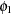and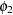: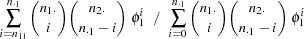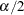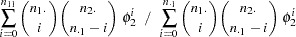When the odds ratio equals zero, which occurs when either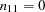or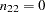, PROC FREQ sets the lower exact confidence limit to zero and determines the upper limit with level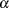. Similarly, when the odds ratio equals infinity, which occurs when either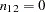or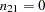, PROC FREQ sets the upper exact confidence limit to infinity and determines the lower limit with level.

#### Relative Risks (Cohort Studies)

These measures of relative risk are useful in cohort (prospective) study designs, where two samples are identified based on the presence or absence of an explanatory factor. The two samples are observed in future time for the binary (yes-no) response variable under study. Relative risk measures are also useful in cross-sectional studies, where two variables are observed simultaneously. See Stokes, Davis, and Koch (2000) and Agresti (2007) for more information.

The column 1 relative risk is the ratio of the column 1 risk for row 1 to row 2. The column 1 risk for row 1 is the proportion of the row 1 observations classified in column 1,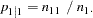Similarly, the column 1 risk for row 2 is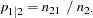The column 1 relative risk is then computed as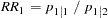A relative risk greater than 1 indicates that the probability of positive response is greater in row 1 than in row 2. Similarly, a relative risk less than 1 indicates that the probability of positive response is less in row 1 than in row 2. The strength of association increases with the deviation from 1.

Asymptotic% confidence limits for the column 1 relative risk are computed as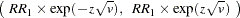where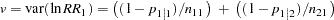andis the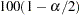th percentile of the standard normal distribution. If either
or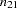is zero, the estimates are not computed.

PROC FREQ computes the column 2 relative risks in the same way.Previous Page | Next Page | Top of Page